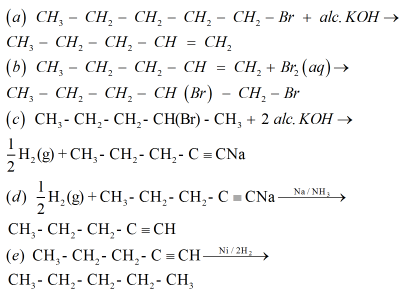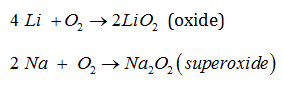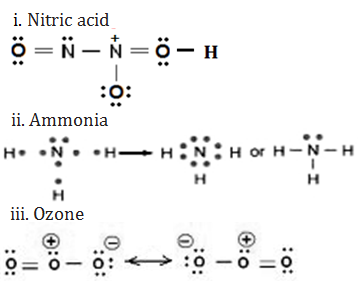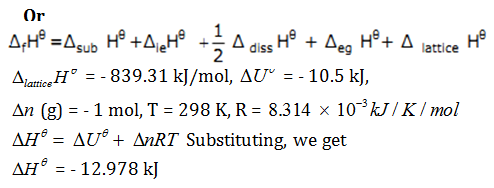# Sample paper for class 11 Chemistry## myCBSEguide App

CBSE, NCERT, JEE Main, NEET-UG, NDA, Exam Papers, Question Bank, NCERT Solutions, Exemplars, Revision Notes, Free Videos, MCQ Tests & more.

Download CBSE sample paper for class 11 Chemistry from myCBSEguide. Sample paper for class 11 Chemistry are available for download in myCBSEguide app, the best app for CBSE students. Sample Paper for class 11 Chemistry includes questions from Chemistry Part-I(Published by NCERT), Chemistry Part-II(Published by NCERT).

For study on the go download myCBSEguide app for android phones. Sample paper for class 11 Chemistry and other subjects are available for download as PDF in app too.

## Chemistry Sample papers

Here is Sample paper for class 11 Chemistry. To get the answers and more sample papers, visit myCBSEguide App or website stated above.

### Sample Paper for class class 11 ChemistrySession 2017-2018

Time allowed: 3 hours, Maximum Marks: 70

General Instructions:
a) All the questions are compulsory.
b) There are 26 questions in total.
c) Questions 1 to 5 are very short answer type questions and carry one mark each.
d) Questions 6 to 10 carry two marks each.
e) Questions 11 to 22 carry three marks each.
f) Questions 23is value based question carrying four marks.
g) Questions 24to 26 carry five marks each.
h) There is no overall choice. However, an internal choice has been provided in one question of two marks, one question of three marks and all three questions in five marks each. You have to attempt only one of the choices in such questions.
i) Use of calculators is not permitted. However, you may use log tables if necessary.

1. What will be the volume of ammonia formed if 2L of nitrogen is mixed with 2L of hydrogen at constant temperature and pressure?
Ans. 1L of nitrogen will react with 3L of hydrogen,
2L of nitrogen will react with 6L of hydrogen, but we have 2L of hydrogen, therefore hydrogen is limiting reactant.
So, 3L of hydrogen gives 2L of ammonia,
2L of hydrogen gives {tex}\frac{\text{2}}{\text{3}}\text{x 2 = }\frac{\text{4}}{\text{3}}\text{ = 1}\text{.33L of ammonia}{/tex}.

2. Give two examples of state functions.
Ans. {tex}\Delta{/tex}H, the enthalpy change and {tex}\Delta{/tex}U, the internal energy change are state functions as they depend on initial and final state and note on the path.

3. Why the molar enthalpy of vapourisation of acetone is less than that of water?
Ans. It is because acetone has weak van der Waals’ forces of attraction whereas water molecules have strong hydrogen bonding, therefore, {tex}\Delta{/tex}vapH0 of water is more.

4. How many electrons are present in 16g of methane?
Ans. 1 molecule of methane = 6 + 4 = 10 electrons
16 of methane contains 10 x 6.022 x 1023 electrons = 6.022 x 1024 electrons.

5. How manygrams of {tex}\mathbf{N}{{\mathbf{a}}_{\mathbf{2}}}\mathbf{C}{{\mathbf{O}}_{\mathbf{3}}}{/tex} should be dissolved to make 100cc of 0.15M {tex}\mathbf{N}{{\mathbf{a}}_{\mathbf{2}}}\mathbf{C}{{\mathbf{O}}_{\mathbf{3}}}{/tex} ?
Ans. 1000 cm3 of 0.15 M {tex}N{{a}_{2}}C{{O}_{3}}{/tex} contains 0.15 moles of Na2CO3
So, 100 cm3 of 0.15 M {tex}N{{a}_{2}}C{{O}_{3}}{/tex} will contain = {tex}\text{= }\frac{\text{0}\text{.15 x 100}}{1000}{/tex}
= 0.015 moles of {tex}N{{a}_{2}}C{{O}_{3}}{/tex}
{tex}\begin{align} & \text{Number of moles = }\frac{\text{Mass}}{\text{Molar mass}} \\ & \therefore \text{0}\text{.015 =}\frac{\text{Mass}}{\text{106g}} \\ \end{align}{/tex}
Molar mass of {tex}N{{a}_{2}}CO{/tex}3 = 106 g
= 0.015 {tex}\times {/tex} 106 = 1.59 g

6. The standard solution of NaOH cannot be prepared by weighing. Why?
Ans. Since NaOH is deliquescent in nature and absorbs both moisture and carbon dioxide from air. The accurate weighing is not possible and not feasible to prepare standard solution of NaOH by weighing.

7. Give reason: “Although geometries of ammonia and water molecules are distorted tetrahedral, bond angle in water is less than that of ammonia”.

Or

Why is benzene extra-ordinary stable though it contains three double bonds?
Ans. In case of ammonia, only one lone pair of electron is present and due to repulsion between lp – bp, the bond angle between bond pairs is 1070. In case of water, two lone pairs of electrons are present. Thus lp – lp repulsion is more than lp – bp. So the bond angle is reduced to 104.50.

Or

The extra-ordinary stability of benzene is due to resonance. Due to this, the π electron cloud gets delocalized resulting in the stability of the molecule.

8. In the estimation of sulphur by Carius method, 0.468 g of an organic sulphur compound afforded 0.668 g of barium sulphate. Find out the percentage of sulphur in the given compound.
Ans. Mass of organic compound = 0.468 g
Mass of {tex}BaS{{O}_{4}}{/tex} formed = 0.668 g
233 g of {tex}BaS{{O}_{4}}{/tex} contains S = 32 g
0.668 of {tex}BaS{{O}_{4}}{/tex} contains S = 32 {tex}\times {/tex} 0.668/233 = 0.917 g
% of S = 0.917/0.468 {tex}\times {/tex} 100 = 19.59%

9. a) Is it possible to achieve equilibrium between water and its vapour in an open vessel?

Ans. No. Here, the rate of evaporation is still constant but since the molecules get dispersed into a large open volume, the rate of condensation from gas to liquid state can never be equal to the rate of evaporates eventually completely.

10. How does electronegativity vary i) down the group and ii) across the period?
Ans. (a)Electronegativity goes on decreasing down in the group due to increase in atomic size.
(b)It goes on increasing along the period due to decrease in atomic size.

11. Give reasons: “The reaction 2 Na(s) + H2 (g) {tex}\to {/tex} 2 NaH (s) is a redox change”.
Ans. Since in the above reaction the compound formed is an ionic compound, which may also be represented as Na+H (s), this suggests that one half reaction in this process is:
2 Na (s) {tex}\to {/tex} 2 Na+(g) + 2e and the other half reaction is: H2 (g) + 2e {tex}\to {/tex} 2 H(g). This splitting of the reaction under examination into two half reactions automatically reveals that here sodium is oxidised and hydrogen is reduced, therefore, the complete reaction is a redox change.

12. Give reason:
(i) Graphite is used as lubricant.
(ii) Diamond is used as an abrasive.
(iii) Aluminium alloys are used to make aircraft body.

Or

Explain isomerization in alkanes with examples.
Ans. (i) Graphite has layered structure in which the different layers are held together by weak Vander Waals forces and hence can be made to slip over one another. So, graphite acts as a lubricant.
(ii) Since diamond is very hard, it can be used as an abrasive.
(iii) Aluminium alloys are light, tough and resistant to corrosion and so are used to make aircraft body.

Or

The n-Alkanes on heating in the presence of anhydrous aluminium chloride and hydrogen chloride gas isomerize to branched chain alkanes. Major products are given below. Some minor products are also possible but are not reported in organic reactions.
CH3(CH2)4CH3 {tex}\xrightarrow{\text{Anhy}\text{.AlC}{{\text{l}}_{\text{3}}}\text{/HCl}}{/tex} CH3-CH(CH3)-(CH2)2-CH3 + CH3-CH2-CH(CH3)-CH2-CH3

13. An alkyl halide compound ‘A’ (C5H11Br) reacts with ethanolic KOH to give compound ‘B’, an alkene. ‘B’ on reaction with bromine gives compound ‘C’. ‘C’ on further dehydrobromination gives compound ‘D’. When one mole of ‘D’ is treated with sodium metal in liquid ammonia, it gives one mole of sodium salt of ‘D’ and half a mole of hydrogen gas. On complete hydrogenation, ‘D’ gives a straight reactions involved.
Ans.14. Calculate the amount of ammonia formed when 50 kg of N2 (g) and 10.0 kg H2 (g) of are mixed to produce NH3 (g). Identify the limiting reagent.
Ans. {tex}\text{Moles of nitrogen = }\frac{\text{Mass}}{\text{Molar mass}}{/tex} = 1.786 x 103 mol
{tex}\text{Moles of hydrogen = }\frac{\text{Mass}}{\text{Molar mass}}{/tex}
= 5.0 x 103 mol
Then, to identify the limiting reagent
{tex}{{\text{N}}_{\text{2}}}\text{(g) + 3}{{\text{H}}_{\text{2}}}(g)\to \text{2N}{{\text{H}}_{\text{3}}}\text{(g) }{/tex}
1 mole of nitrogen gas reacts with 3 moles of hydrogen gas
Therefore 1.786 x 103 mol of nitrogen will react with {tex}\frac{\text{3 x 1}\text{.786 x 1}{{\text{0}}^{3}}}{\text{1}}{/tex} moles of hydrogen
= 5.36 {tex}\times {/tex} 103 mol
Since, there is 5.0 x 103 mol of hydrogen only, it is the limiting reagent.
To calculate the amount of ammonia formed,
3 moles of hydrogen gives 2 moles of ammonia,
Therefore 5.0 x 103 moles of hydrogen gives {tex}\frac{2}{3}\text{ x 5 x 1}{{\text{0}}^{3}}{/tex} of ammonia = 3.3 {tex}\times {/tex}103 moles of ammonia.
Mass of NH3 produced = 3.3 {tex}\times {/tex}103 {tex}\times {/tex} 17 g of {tex}N{{H}_{3}}{/tex} = 56.1 kg

15. Give reasons:
i. Evaporation causes cooling.
ii. Falling liquids drops are spherical.
iii. Vapour pressure of acetone is less than that of ether at same temperature.

Ans. (i) This is due to the reason that the molecules which undergo evaporation are high energy molecules and therefore, the kinetic energy of the remaining molecules becomes less. Since the remaining molecules have lower average kinetic energy, their temperature becomes low.
(ii) This is due to surface tension of liquids. Due to surface tension, the molecules of a liquid, try to make surface area to be minimum and for a given volume, sphere has the minimum surface area. Therefore the falling liquid drops are spherical.
(iii) Intermolecular forces are stronger in acetone than in ether. Thus the vapour pressure of acetone is less than ether.

16. What happens when alkali metals reacts in air?
Ans. The alkali metals tarnish in dry air due to the formation of their oxides which in turn react with moisture to form hydroxides. They burn vigorously in oxygen forming oxides. Lithium forms monoxide, sodium forms peroxide, the other metals form super oxides. The superoxide O2– ion is stable only in the presence of large cations such as K, Rb, Cs.17. Give the Lewis representation of:
i. Nitric acid
ii. Ammonia
iii. Ozone molecule

Ans.18. In the reaction: {tex}\text{2S}{{\text{O}}_{\text{2}}}\text{(g) + }{{\text{O}}_{\text{2}}}\to \text{2S}{{\text{O}}_{\text{3}}}\text{(g) + 189}\text{.4 kJ}{/tex}
Indicate the direction in which the equilibrium will shift when:
i) Concentration of SO2 is increased.
ii) Concentration of SO3 is increased.
iii) Temperature is increased.

Ans. (i) If the concentration of SO2 is increased the equilibrium will shift in the forward direction to consume the reactant SO2.
(ii) If the concentration of SO3 is increased the equilibrium will shift in the backward direction to consume the product SO3.
(iii) If the temperature is increased, the equilibrium will shift in the backward direction as the increase in temperature will be compensated by absorbing heat

19. Calculate the wavelength in nm, of visible light having a frequency of {tex}4.37\text{ }\times \text{ }{{10}^{-14}}/s.{/tex}
Ans. Since, {tex}\nu =\frac{c}{\lambda }{/tex}
Substituting the values,
Λ = 686 nm
Here, n1 = 6 and n2 = 1,
The energy gap between two orbits for a hydrogen atom is given as,
{tex}\Delta{/tex}E = {tex}\text{2}\text{.18 x 1}{{\text{0}}^{\text{-18}}}\left( \frac{\text{1}}{\text{n}_{\text{1}}^{\text{2}}}\text{-}\frac{\text{1}}{\text{n}_{\text{2}}^{\text{2}}} \right){/tex}
{tex}=\text{ }-\text{ }2.11\text{ }\times \text{ }{{10}^{-18}}J~{/tex}
Since {tex}\Delta{/tex}E is negative energy, the frequency of photon is given by
{tex}\nu =\frac{\Delta E}{h}{/tex}
Substituting, we get
{tex}\text{ }\!\!\nu\!\!\text{ = 3}\text{.18 x 1}{{\text{0}}^{\text{15}}}\text{ Hz}{/tex}

20. Comment on each of the following observations:
a) Lithium forms a nitride directly like magnesium. Give equation involved.
b) BaO is soluble but {tex}BaS{{O}_{4}}{/tex} is insoluble in water.

Ans. (a) Lithium and magnesium follow diagonal relationship and so lithium like magnesium forms nitride while other alkali metals do not.
(b)Size of {tex}{{O}^{2-}}{/tex} ion is smaller than {tex}S{{O}_{4}}^{2-}.{/tex} Since a bigger anions stabilizes bigger cation more than a smaller cation stabilizes a bigger anion, lattice enthalpy of BaO is smaller than BaSO4. BaO is soluble as hydration energy is more than lattice energy but {tex}~BaS{{O}_{4}}{/tex} (as hydration energy is less than lattice energy) is insoluble in water.

21. Give a note on:
i. Mist
ii. Smoke
iii. Fumes
iv. Dust

Ans. (i) Mists are formed when certain herbicides and insecticides are sprayed in the liquid form over the plants.
(ii)Smokes are small particles of soot which are released in atmosphere in the form of oil, smoke etc by incomplete combustion of organic matter.
(iii)Fumes are released to atmosphere in metallurgical operations due to result of reactions in factories.
(iv)Dusts are released by grinding limestone, cement as fly ash etc.

22. Calculate the enthalpy change when 2.38g of CO vaporizes at its normal boiling point, if the enthalpy of vaporization of CO is 6.04 kJ/mol.
Ans. Enthalpy of vaporization of CO = 6.04 kJ/mol
Molar mass of CO = 28 g/mol
Enthalpy change for vaporization of 28 g of CO at boiling point = 6.04 kJ
Therefore, the enthalpy change for vapourization of 2.38 g of CO at boiling point will be,
{tex}\frac{\text{6}\text{.04 x 2}\text{.38}}{\text{28}}\text{=0}\text{.5134kJ}{/tex}
= 513.4 J

23. John takes snacks every day to school, but Mala takes vegetables, chapattis and curd. Chips and snacks packet are filled with nitrogen gas. If they are filled with oxygen, they will get rancid.
a) What is meant by rancidity?
b) How do you preserve butter?
c) Why chips are packed with nitrogen gas?

Ans. (a)The spoilage of food due to oxidation is called rancidity.
(b) We preserve butter at low temperature. Adding common salt and antioxidants to prevent it from getting spoiled.
(c) It is done to prevent it from oxidation.

24. Three students, A, B and C were asked to prepare the Lassaigne’s extract independently by fusing the compound with sodium. Then, they added solid ferrous sulphate and dilute sulphuric acid to a part of Lassaigne’s extract. Both A and B got Prussian blue colour but C got red colour. Can you help them with equations and reasons? Write the chemical equations to explain the formation of compounds of different colours.

Or

a) What is the principle of chromatography?
b) How can forgery be detected with the help of chromatography?
c) Is it possible to separate components of orange ink by chromatography?
d) Name the stationary and mobile phase in paper chromatography.
e) What is the suitable adsorbent in the process of column chromatography?

Ans. If S and N both are present, it result in the formation of NaSCN
{tex}Na\text{ }+\text{ }S\text{ }+\text{ }C\text{ }+\text{ }\to NaSCN{/tex}
It also reacts with Fe3+ and gives red colour due to the formation of ferric thiocyanate.
{tex}F{{e}^{3+}}+\text{ }SC{{N}^{-}}\to {{\left[ Fe\left( SCN \right) \right]}^{2+}}{/tex}
This takes place when fusion is not carried out in excess of sodium. If excess of sodium is used, then thiocyanate ion will change to NaCN and sodium cyanide.
{tex}NaSCN\text{ }+\text{ }2Na\to NaCN\text{ }+\text{ }N{{a}_{2}}S{/tex}
The Prussian blue colour is formed due to the formation of ferric ferro cyanide.
{tex}F{{e}^{2+}}+\text{ }6C{{N}^{-}}\to {{\left[ Fe{{\left( CN \right)}_{6}} \right]}^{4-}}{/tex}

Or

a) It is based on the principle of differential adsorption.
b) Different inks have different adsorbing power; therefore it can be detected by chromatography.
c) Yes.
d) Liquid on paper is stationary phase and the solution of substance to be separated is mobile phase.
e) Alumina.

25. Give reasons:
a) Why silicones are used for nipples of feeding bottles?
b) Why are silicones used in cosmetic plants?
c) Why silicones are water-repellant?
d) Why are silicones thermally stable?
e) Are silicones safe for environment?

Or

i) If a salt ‘A’ gives the following results:
a) Its aqueous solution is alkaline to litmus.
b) On strong heating, ‘A’ swells up to a glassy material ‘B’.
c) When concentrated HCl is added to a hot solution of ‘A’ white crystals of an acid ‘C’ separates out.
Write the chemical equations for the reactions and identify ‘A’, ‘B’ and ‘C’.
ii) Complete the equations:
a) 2Al + 6HCl {tex}\to {/tex}
b) 8BF3 + 6LiH {tex}\to {/tex}

Ans. (a) Because it is thermally stable.
(b)Because they are biocompatible.
(c)Since they are surrounded by non-polar alkyl groups which is water repelling.
(d)Because they are highly polar and have strong forces of attraction.
(e)Yes.

Or

i) ‘A’ is borax {tex}\left( N{{a}_{2}}{{B}_{4}}{{O}_{7}}.10{{H}_{2}}O \right){/tex}
a) The aqueous solution of borax is alkaline to litmus.
{tex}N{{a}_{2}}{{B}_{4}}{{O}_{7}}+\text{ }2{{H}_{2}}O\to 2NaOH\text{ }+\text{ }{{H}_{2}}{{B}_{4}}{{O}_{7}}{/tex}
b) Borax swells to a glassy material on strong heating to give sodium metaborate, ‘B’
{tex}\text{N}{{\text{a}}_{\text{2}}}{{\text{B}}_{\text{4}}}{{\text{O}}_{\text{7}}}\xrightarrow{\text{1000K}}\text{2NaB}{{\text{O}}_{\text{2}}}\text{+ }{{\text{B}}_{\text{2}}}{{\text{O}}_{\text{3}}}{/tex}
c) On adding hot solution of ‘A’ to conc. HCl, it gives boric acid, ‘C’.
Na2B4O7 + 2HCl + 5H2O {tex}\to {/tex} 2NaCl + 4H3BO3
ii) a) {tex} 2Al\text{ }+\text{ }6HCl\to 2AlC{{l}_{3}}+\text{ }3{{H}_{2}}{/tex}
b) {tex} 8B{{F}_{3}}+\text{ }6LiH\to {{B}_{2}}{{H}_{6}}+\text{ }6LiB{{F}_{4}}{/tex}

26. i) What is the change in internal energy in a process, 701 J of heat is absorbed by a system and 394 J of work is done by the system.
ii) The equilibrium constant for the reaction is 10. Calculate the value of {tex}\Delta{/tex}G{tex}\theta{/tex}. Given R = 8.0 J/mol, T = 300 K.

Or

Calculate the lattice energy for the change of {tex}L{{i}^{+}}\left( g \right)\text{ }+\text{ }C{{l}^{-}}\left( g \right)\to LiCl\left( s \right),{/tex}
{tex}{{\Delta }_{sub}}{{H}^{\theta }}{/tex} of Li = 160.67 kJ/mol, {tex}{{\Delta }_{diss}}{{H}^{\theta }}{/tex} of Cl2 = 244.34 kJ/mol,
{tex}{{\Delta }_{le}}{{H}^{\theta }}{/tex}of Li (g) = 520.07 kJ/mol, {tex}{{\Delta }_{eg}}{{H}^{\theta }}{/tex} of Cl (g) = – 365.26 kJ/mol,
{tex}\Delta_{r}{{H}^{\theta }}{/tex} of LiCl (s) = – 401.66 kJ/mol.
Is the reaction spontaneous or not?

Ans. (i) Heat absorbed by the system (q)= + 701 J
Work done by the system (w) = – 394 J
Change in the internal energy ({tex}\Delta{/tex}U) = q + w = + 701 + ( – 394) = + 307 J
(ii) {tex}\Delta {{G}^{\theta }}{/tex} = -2.303 RT log K
Substituting, the values, we get = – 5527.2 J/molSubstituting the values for {tex}\Delta {{G}^{\theta }}=\text{ }\Delta {{H}^{\theta }}-\text{ }T\Delta {/tex} S{tex}\theta{/tex}, we get
{tex}\Delta {{G}^{\theta }}{/tex} = – 2816.2 J
Since the value of {tex}\Delta {{G}^{\theta }}{/tex} is negative, the reaction is spontaneous.

This is only initial part of the whole sample paper. Download Complete set of sample paper for class 11 Mathematics

## Sample Paper for class 11

It is Sample paper for class 11 Chemistry. However, myCBSEguide provides the best sample papers for all the subjects. There are number of sample papers which you can download from myCBSEguide website. Sample paper for class 11 all subjects

These are also Sample Paper for class 11 Chemistry available for download through myCBSEguide app. These are the latest Sample Paper for class 11 Chemistry as per the new exam pattern. Download the app today to get the latest and up-to-date study material.

## Marking Scheme for Class 11 exam

 Subject Board Marks Practical or internal Marks English 100 Marks ZERO Marks Hindi 100 Marks ZERO Marks Mathematics 100 Marks ZERO Marks Chemistry 70 Marks 30 Marks Physics 70 Marks 30 Marks Biology 70 Marks 30 Marks Computer Science 70 Marks 30 Marks Informatics Practices 70 Marks 30 Marks Accountancy 80 Marks 20 Marks Business Studies 80 Marks 30 Marks Economics 80 Marks 20 Marks History 80 Marks 20 Marks Political Science 100 Marks ZERO Marks Geography 70 Marks 30 Marks Sociology 80 Marks 20 Marks Physical Education 70 Marks 30 Marks Home Science 70 Marks 30 Marks